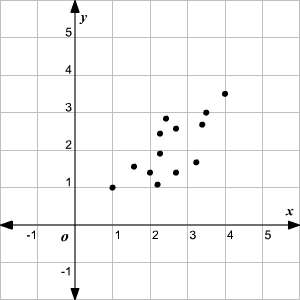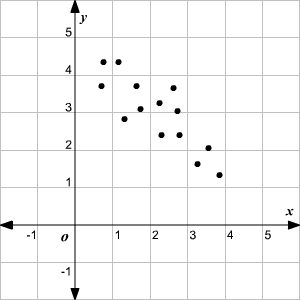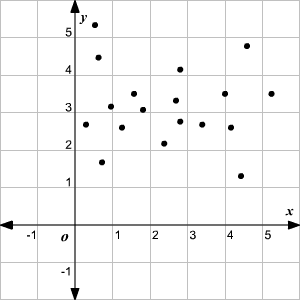# Correlation and Causal Relation

A correlation is a measure or degree of relationship between two variables. A set of data can be positively correlated, negatively correlated or not correlated at all. As one set of values increases the other set tends to increase then it is called a positive correlation.As one set of values increases the other set tends to decrease then it is called a negative correlation.If the change in values of one set doesn't affect the values of the other, then the variables are said to have "no correlation" or "zero correlation."A causal relation between two events exists if the occurrence of the first causes the other. The first event is called the cause and the second event is called the effect. A correlation between two variables does not imply causation. On the other hand, if there is a causal relationship between two variables, they must be correlated.

Example:

A study shows that there is a negative correlation between a student's anxiety before a test and the student's score on the test. But we cannot say that the anxiety causes a lower score on the test; there could be other reasons—the student may not have studied well, for example. So the correlation here does not imply causation.

However, consider the positive correlation between the number of hours you spend studying for a test and the grade you get on the test. Here, there is causation as well; if you spend more time studying, it results in a higher grade.

One of the most commonly used measures of correlation is Pearson Product Moment Correlation or Pearson's correlation coefficient. It is measured using the formula,The value of Pearson's correlation coefficient vary fromtowhere –1 indicates a strong negative correlation andindicates a strong positive correlation.